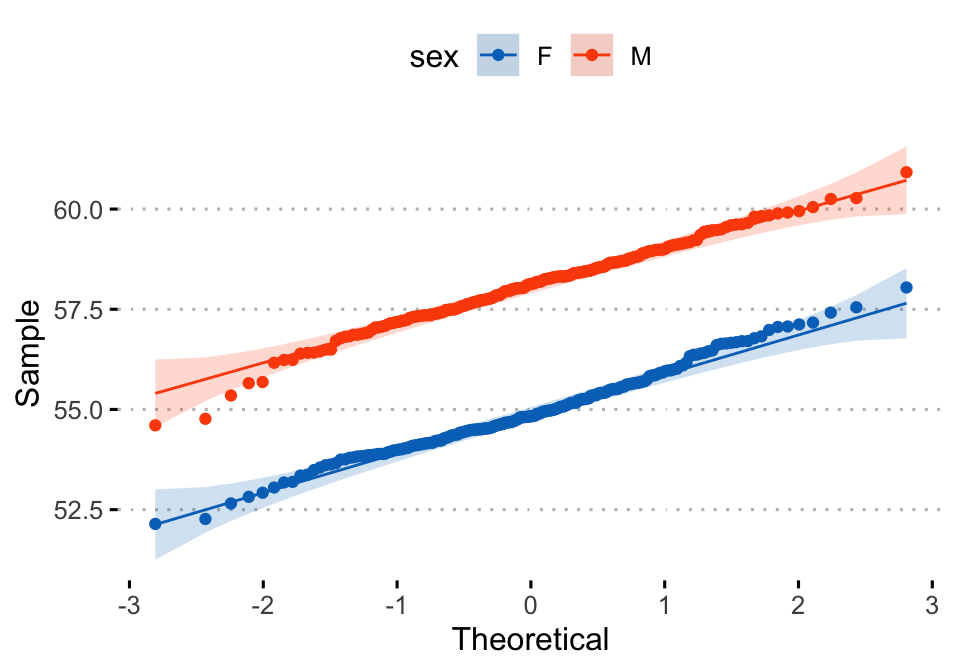# Data Visualization using GGPlot2

## GGPLOT QQ Plot

A Quantile-quantile plot (or QQPlot) is used to check whether a given data follows normal distribution.

The data is assumed to be normally distributed when the points approximately follow the 45-degree reference line.

This article describes how to create a qqplot in R using the ggplot2 package.

Contents:

#### Related Book

GGPlot2 Essentials for Great Data Visualization in R

## Key R functions

• Key function: stat_qq().
• Key arguments: color, shape and size to change point color, shape and size.

## Data preparation

Create some data (wdata) containing the weights by sex (M for male; F for female):

set.seed(1234)
wdata = data.frame(
sex = factor(rep(c("F", "M"), each=200)),
weight = c(rnorm(200, 55), rnorm(200, 58))
)

# head(wdata, 4)

Load the ggplot2 package and set the default theme to theme_minimal() with the legend at the top of the plot:

library(ggplot2)
theme_set(
theme_minimal() +
theme(legend.position = "top")
)

## Create qqplots

Create a qq-plot of weight. Change color by groups (sex)

ggplot(wdata, aes(sample = weight)) +
stat_qq(aes(color = sex)) +
scale_color_manual(values = c("#00AFBB", "#E7B800"))+
labs(y = "Weight")Alternative plot using the function ggqqplot() [in ggpubr]. The 95% confidence band is shown by default.

library(ggpubr)
ggqqplot(wdata, x = "weight",
color = "sex",
palette = c("#0073C2FF", "#FC4E07"),
ggtheme = theme_pubclean())## Conclusion

This article shows how to create a qqplot using the ggplot2 and the ggpubr package.